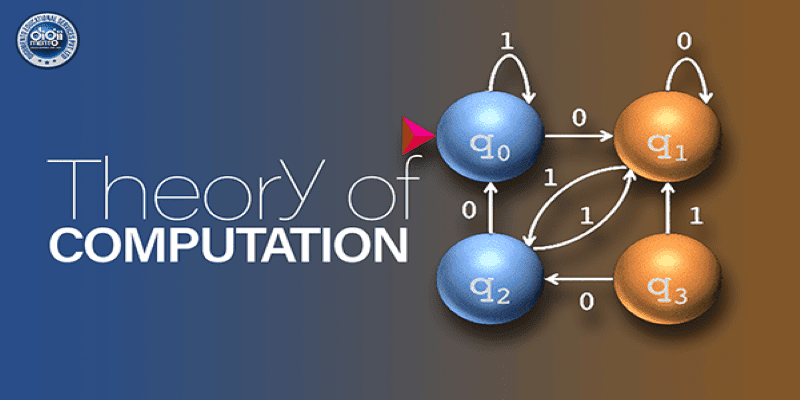# Theory of Computation part 1#### The theory of computation is a branch of computer science that studies the ability of computers to solve problems. It is a mathematical field of study that focuses on the power and limitations of computing devices. In other words, it is concerned with the study of what computers can and cannot do. The theory of computation is based on the idea that any problem can be solved if it is broken down into smaller problems and each problem is solved one at a time. This approach is known as an algorithm, which is a sequence of instructions that can be followed to solve a given problem. Algorithms are used in computer programming to create software applications. The most important concept in the theory of computation is the Turing machine, which is a hypothetical machine that can solve any computable problem. This machine is used to prove theorems and to determine the computational power of computers. The theory of computation also studies the complexity of algorithms. Complexity refers to the amount of time and resources needed to solve a given problem. Algorithms that require more time and resources are said to be more complex than those that require less. The theory of computation is also used to study the limits of what computers can do. For example, some problems may be too difficult to solve efficiently and require too much time to compute. In these cases, computers might not be able to solve them and we must look for alternative solutions. The theory of computation is a fascinating field that has many applications in the real world. It is used to create more efficient algorithms and solve complex problems. It is also a powerful tool for understanding the power and limitations of computers.

This is an introductory course on the theory of computation intended for undergraduate students in computer science. In this course, we will introduce various models of computation and study their power and limitations. We will also explore the properties of corresponding language classes defined by these models and the relations between them. It is designed based on the syllabus given by the GATE Computer Science exam.

The Course contains a formal connection between algorithmic problem solving and the theory of languages, automata. It also develops them into a mathematical (and less magical) view towards the algorithmic design and in general computation itself. The course should, in addition, clarify the practical view towards the applications of these ideas in the engineering part of CS.

Who this course is for:

1. Anyone who is interested in learning the theory of computation and its concepts.

### What Will You Learn?

• One can master Finite Automata of Theory of Computation
• In-depth understanding of the basics of the Theory of Computation concepts
• Understanding the need to study the theory of computation
• Understanding the advanced concepts of the theory of computation like Push Down Automata

07:25
07:48
12:09
10:17
08:16
17:29
15:07
11:11
14:52
14:38
18:31
16:50
07:37
14:57
00:00
00:00
00:00
00:00
00:00
00:00
00:00
10:31
11:22
00:00
00:00
00:00
00:00
00:00
00:00
00:00
00:00
00:00
00:00
00:00
00:00

### Student Ratings & ReviewsNo Review Yet